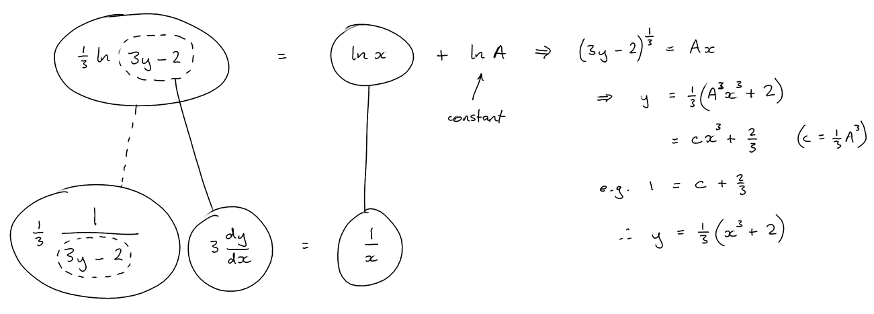# Thread: Differential Equations

1. ## Differential Equations

Hi,
can anyone help with this probem:

Solve the following differential equations subject to the stated condition on y(x)x(dy/dx)-3y+2=0 when y(1)=12. ## Re: Differential Equations

Separate...

$\displaystyle x\ \frac{dy}{dx}\ -\ 3 y + 2 = 0$

$\displaystyle \Rightarrow\ \frac{1}{3 y - 2}\ \frac{dy}{dx}\ =\ \frac{1}{x}$

Spoiler:

Just in case a picture helps...... where (key in spoiler) ...

Spoiler:... is the chain rule. Straight continuous lines differentiate downwards (integrate up) with respect to the main variable (in this case x), and the straight dashed line similarly but with respect to the dashed balloon expression (the inner function of the composite which is subject to the chain rule).

_________________________________________

Don't integrate - balloontegrate!

Balloon Calculus; standard integrals, derivatives and methods

Balloon Calculus Drawing with LaTeX and Asymptote!

3. ## Re: Differential Equations

Thank you tom@balooncalculus! Just wondering, what does the 'A' stand for? If I were looking just for C, would that be 'C=1/3' ?
Regards
NettieL

4. ## Re: Differential Equations

Pleasure. I was taught the habit of using ln A as the constant of integration where it might save having to switch to a different name for the constant part of the solution. But in this case I did end up having to switch from A to another, so using the log constant at first probably didn't help at all.

Anyway, when you say you're looking just for c, well if you mean to identify the constant term in the particular solution, that term is 2/3. Whereas 1/3 is the co-efficient of x^3.

On the other hand, c was the unknown constant in the general solution. (And we used the stated condition to pin it down.)

#### Search Tags

differential, equations